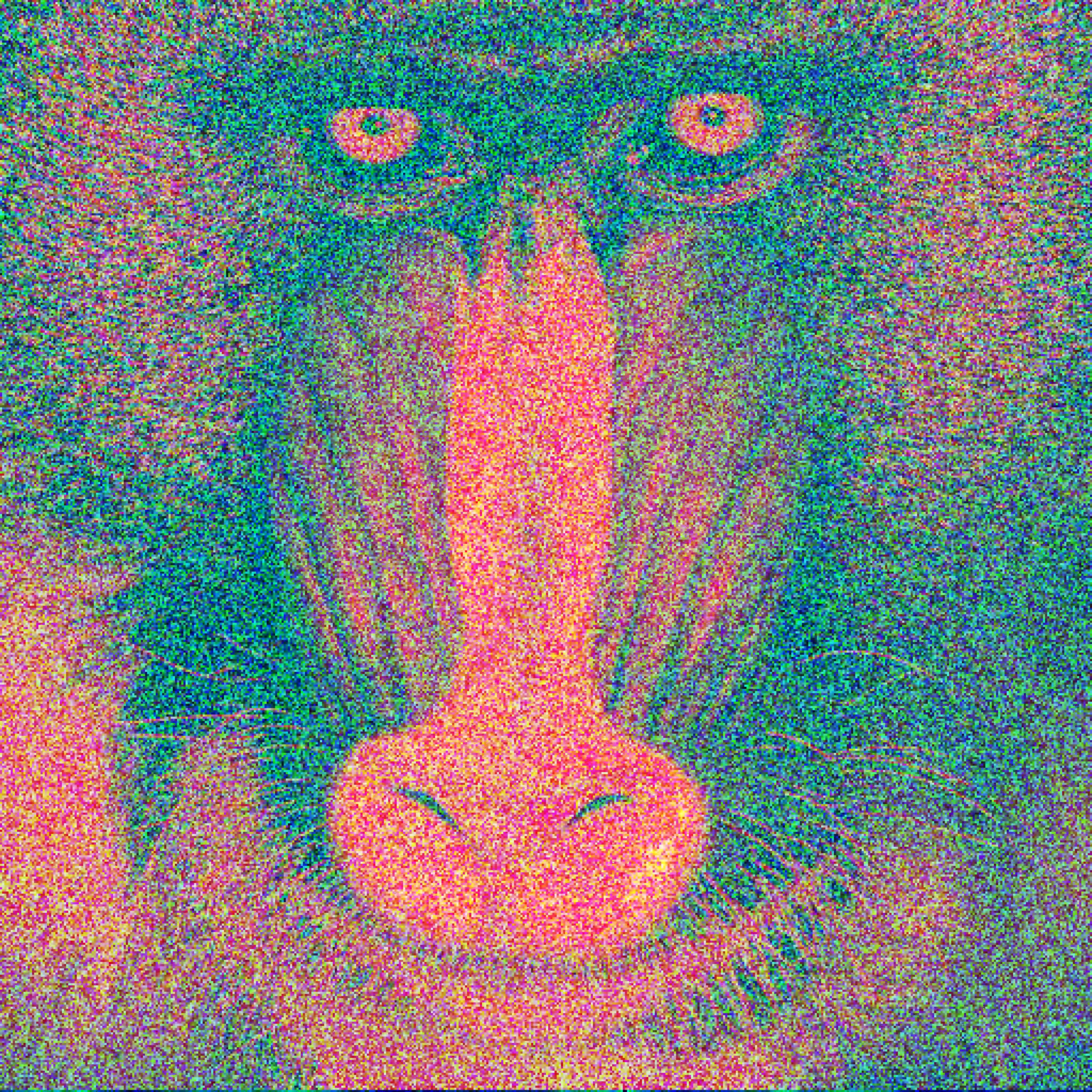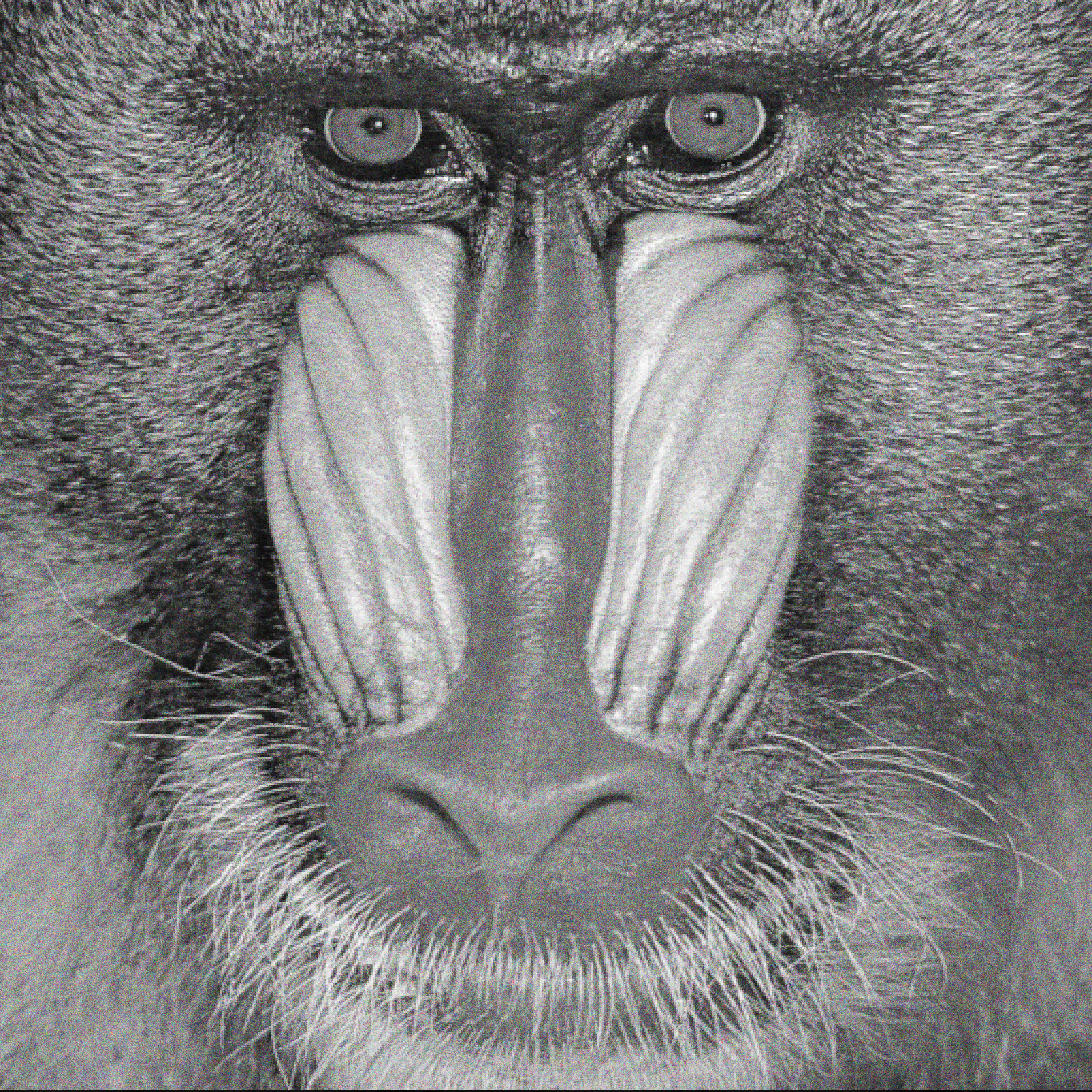# Colour Sort

Date: 2020-06-24

## What is an allRGB image?

I first encountered the concept when I stumbled across allrgb.com.

The objective of allRGB is simple: To create images with one pixel for every RGB color (16,777,216); not one color missing, and not one color twice.

As I assume would be the case for most developers, after the idea was presented to me I started to contemplate how I might go about transforming an image such that it meets that criteria but remains recognizable.

## colour_sort

Find the code On my GitHub.

### Technique

The thing that caught my interest about the subject was that I almost immediately came up with a technique that I figured might work, but honestly I had no idea until I put together a proof of concept.

The idea is simple enough that I can just show you an MVP:

``````import numpy as np
from PIL import Image

# Create a 4096x4096 version of the input image, then squash it down to a single row
im = Image.open("examples/mandrill.tiff").convert("RGB")
resized = im.resize((4096, 4096))
src = np.reshape(np.array(resized), (4096*4096, 3))

# Generate a row containing every RGB colour (Our result array)
red, green, blue = np.arange(256, dtype='u1'), np.arange(256, dtype='u1'), np.arange(256, dtype='u1')
res = np.array(np.meshgrid(red, green, blue)).T.reshape(-1, 3)

# Split the two images into their red, green, and blue components
res_r, res_g, res_b = res.astype(np.uint32).transpose()
src_r, src_g, src_b = src.astype(np.uint32).transpose()

# Combine all three values into 1 to use in sorting later
combined_res = (res_r << 16) | (res_g << 8) | res_b
combined_src = (src_r << 16) | (src_g << 8) | src_b

# Sort the result pixels (By red, then green, then blue due to how they were combined) 
res_sorted = res[np.argsort(combined_res)]

# Generate a mapping of unsorted source image -> sorted source image
mapping = np.argsort(combined_src)

# Reverse that mapping so that it is sorted souce image > source image
reverse_mapping = np.argsort(mapping)

# reverse the sorting on the sorted result image as if it was the sorted source image
final_result = res_sorted[reverse_mapping]

# Turn the image back into 4096x4096 and save it
image = Image.fromarray(np.reshape(final_result, (4096, 4096, 3)), mode='RGB')
image.save('examples/mandrill-sorted.webp')``````
•  This will come up later, but the important part is that the step to generate a “critera” version of the result can be switch out with other methods (For example switching the order of presidence for red, green, and blue) to produce different end results.

The general idea is as follows:

1. Generate an image containing every RGB value exactly once, and sort it by some criteria (In the example above they are sorted by red, then green, then blue values). We will call this the result image.
2. Generated a mapping to sort the source image by the same criteria. This mapping is then used to generate the reverse mapping; That is how to take the sorted version and turn it back into the orignal source image.
3. This mapping is then instead used to “unsort” the result image. The output of that is our final image.Figure 1: MandrillFigure 2: Not the greatest reproduction, but it is recognizable.

With the concept at least showing that it could work, I started experimenting with different strategies by which the pixels should be sorted.

It turns out that red, then green, then blue is rarely a good strategy.

### Examples

Note: If you actually downloaded and tested the images embeded in this page, you’d find that they actually do not contain all RGB values uniquely, but this is just because they have been compressed to reduce the page weight. (You can download full examples here)[https://www.dropbox.com/sh/gjp1wsf8ubvzfl8/AAD27FD_RgjjItuyK3eMoFdca?dl=0].Figure 3: This example is likely the best of the methods I ran the mandrill image through. It sorts the images by blue, red, then green with a twist that part of the computation is purposly allowed to overflow. Originally this strategy was a bug with a previous implementation, but after looking at the results I noticed that it often produced nice looking images, so I decided to keep the bugged behavior as one of the options.Figure 4: It’s a bit boring, but a method that almost always produces a good looking image is to take the average value of the red, green, and blue value for each pixel and use that as the criteria by which the lists are sorted.

### Verification

As part of writing this project I also wrote a verification function that confirms if an image is a valid allRGB image or not.